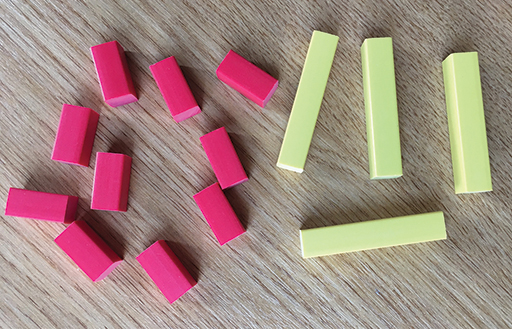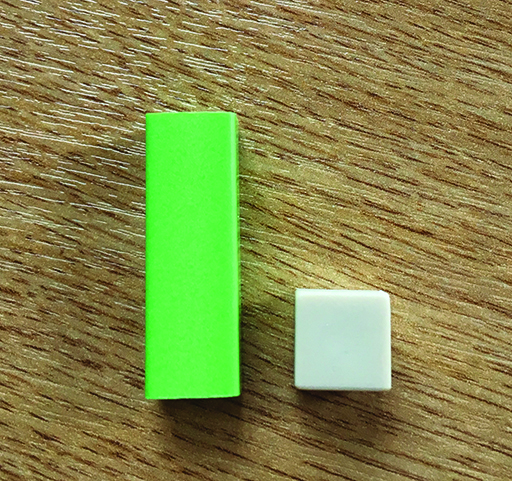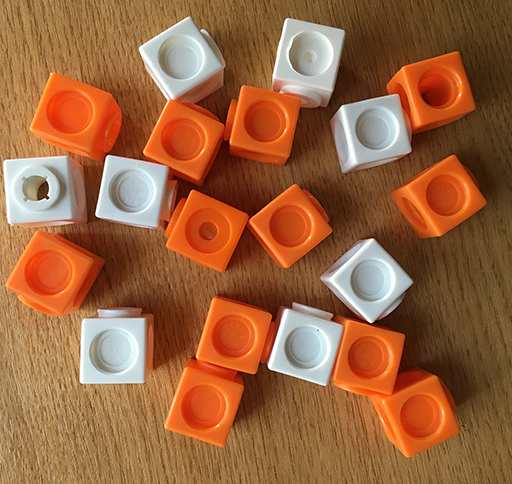Teaching mathematics

Start this free course now. Just create an account and sign in. Enrol and complete the course for a free statement of participation or digital badge if available.

Free course

# 3.1 Using simple ratios to make comparisons

First, look at Figures 20–22 and then do the activity based on them.Figure 20 Using Cuisenaire rods to represent ratiosFigure 21 Using Cuisenaire rods to compare the ratio of lengthsFigure 22 Using multilink cubes to represent ratios

## Activity 18 Writing ratios

Timing: Allow 5 minutes

Write the ratios for each of the images shown above in the simplest form.

• a.What is the ratio of red rods to yellow rods?
• b.What is the ratio of the lengths of the green and white rods?
• c.What is the ratio of orange to white cubes?

### Discussion

• a.10:4 which simplifies to 5:2.
• b.3:1.
• c.12:8 which simplifies to 3:2.
TM_1